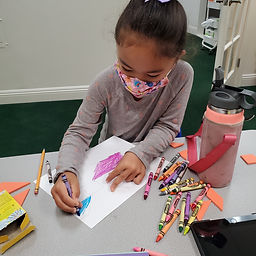## Ms. Mari

### Target 1​

###### Lesson Type:

Continuation

Geometry

:

Modeling

Visualize spatial relationships between shapes and planar space.

###### 1:

Solve simple tangram puzzles by recognizing which spaces in the puzzle the given seven shapes can and cannot fit.

###### 2:

Recognize and apply reflections (flips), translations (slides), and rotations (turns).

2nd

###### Vocabulary:

*Tangrams, Triangles, Squares, & Parallelogram
*Slides, Flips, & Turns

Activities:

• Students identified shapes in a tangram. Parallelogram was introduced.
• Students used tangrams to develop problem solving skills.
• Students flipped and turned shapes to create a rabbit with their tangrams.
• Students flipped, slid, and turned 1 tan (tangram piece) to create a picture.
• Students identified which transformation was used - slide, flip, or turn.### Home Exploration

###### Guiding Questions:## Absent Students:

### Target 2

:

###### 1:

Identify the Commutative Property of Multiplication.

3rd

###### Vocabulary:

Commutative Property, Product, Array

Activities:

• Students completed a table solved equations (ex. 6x4). Table included - a representation, an array (rectangular arrangement in rows & columns), commutative property, product.
• Students learned that the commutative property of multiplication says that the order of the numbers can be changed when multiplying.
• We discussed how (when using the commutative property) the numbers are the same, but the number order was switched. The product remains the same.### Home Exploration

###### Guiding Questions:### Target 3

:

###### Vocabulary:

Activities:### Home Exploration